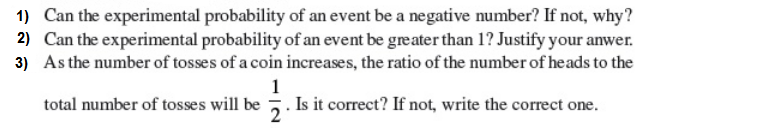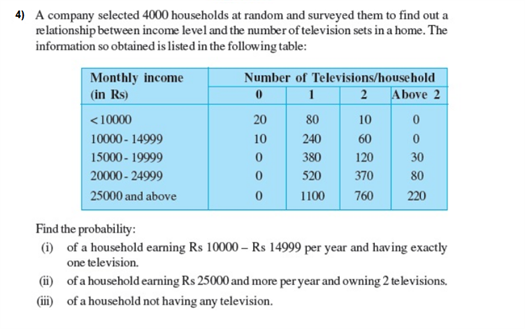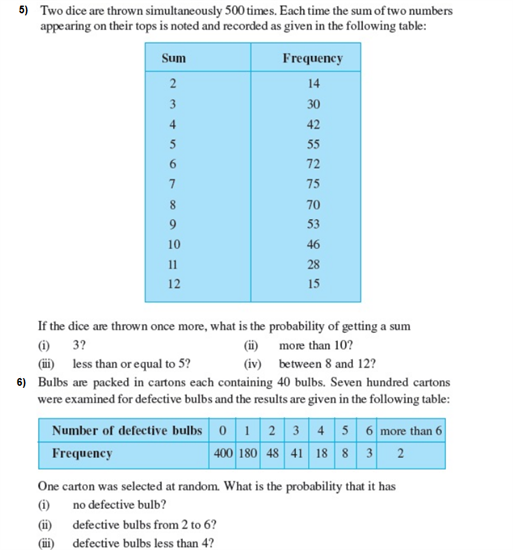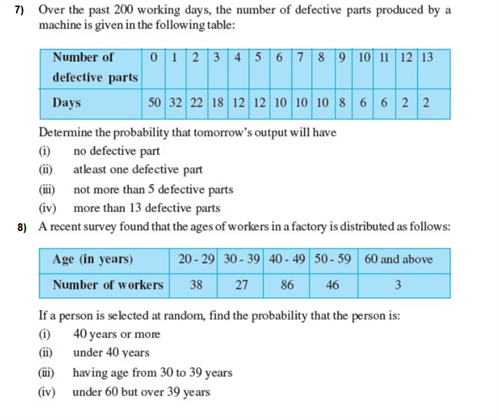# Important Questions Class 9 Maths Chapter 15 Probability

Important class 9 maths questions for chapter 15 Probability are provided here which will help the CBSE students to get a deeper understanding of the concepts. These class 9 extra questions from probability chapter (NCERT) includes various short answer type questions and long answer type questions along with HOTS questions.

Also Check:

## Important Probability Questions for Class 9- Chapter 8 (With Solutions)

1. Compute the probability of the occurrence of an event if the probability the event not occurring is 0.56.

Solution:

Given,

P(not E) = 0.56

We know,

P(E) + P(not E) = 1

So, P(E) = 1 – P(not E)

P(E) = 1 – 0.56

Or, P(E) = 0.44

2. In a factory of 364 workers, 91 are married. Find the probability of selecting a worker who is not married.

Solution:

Given,

Total workers (i.e. Sample space) = S = 364

Total married workers = P(married) = 91

Now, total workers who are not married = (E) = 364 – 91 = 273

Method 1: So, P(not married) = E/S = 273/364 = 0.75

Method 2: P(married) + P(not married) = 1

Here, P(not married) = 91/364 = 0.25

So, 0.25 + P(not married) = 1 – 0.25 = 0.75

3. From a deck of cards, 10 cards are picked at random and shuffled. The cards are as follows:

6, 5, 3, 9, 7, 6, 4, 2, 8, 2

Find the probability of picking a card having value more than 5 and find the probability of picking a card with an even number on it.

Solution:

Total number of cards = 10

Total cards having value more than 5 = 5 (6, 9, 7, 6, 8)

Total cards having an even number = 6 (6, 6, 4, 2, 8, 2)

So, the probability of picking a card having value more than 5 = 5/10 = 0.5

And, the probability of picking a card with an even number on it = 6/10 = 0.6

4. From a bag of red and blue balls, the probability of picking a red ball is x/2. Find “x” if the probability of picking a blue ball is ⅔.

Solution:

Here, there are only red and blue balls.

So, the probability of picking a blue ball = probability of not picking a red ball.

P(picking a red ball) + P(not picking a red ball) = 1

x/2 + ⅔ = 1

=> 3x + 4 = 6

=> 3x = 2

Or, x = ⅔

5. Two coins are tossed simultaneously for 360 times. The number of times ‘2 Tails’ appeared was three times ‘No Tail’ appeared and the number of times ‘1 tail’ appeared is double the number of times ‘No Tail’ appeared. What is the probability of getting ‘Two tails’.

Solution:

Given,

Total number of outcomes = Sample space = 360

Now, assume that the number of times ‘No Tail’ appeared to be “x”

So, the number of times ‘2 Tails’ appeared = 3x (from the question)

Also, the number of times ‘1 Tail’ appeared =2x (from the question)

As the total outcomes = 360,

x + 2x + 3x = 360

=> 6x = 360

Or, x = 60

∴ P(getting two tails) = (3 × 60)/360 = ½

### Extra Questions For Class 9 Chapter 15: Probability (NCERT)